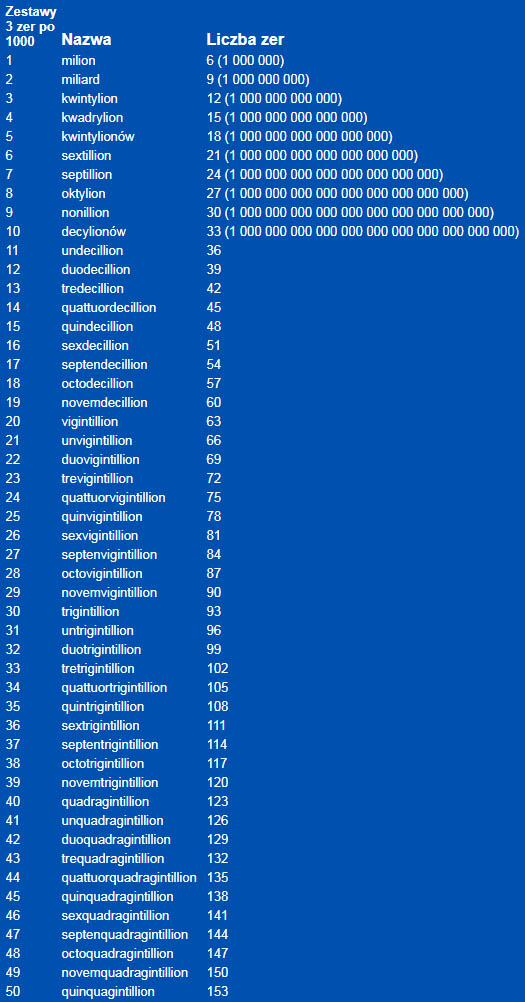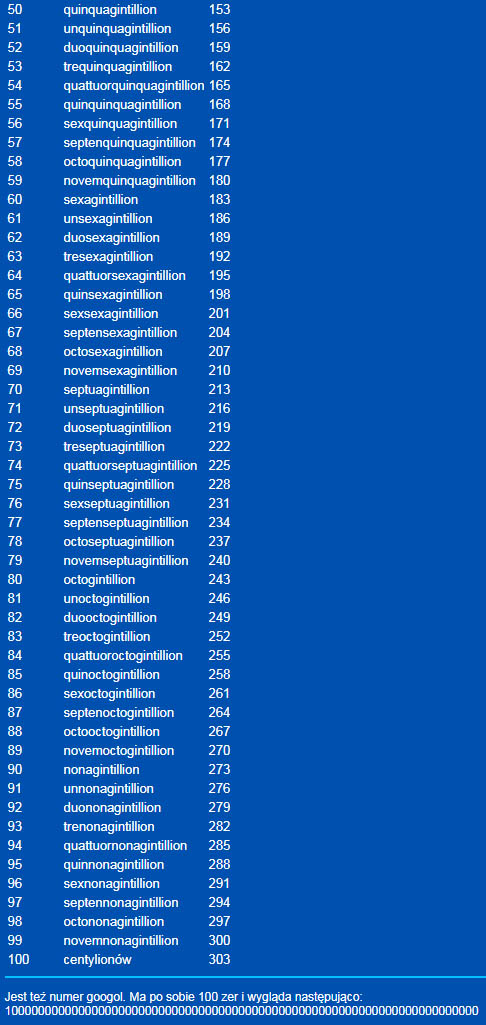# the biggest number

#### What is the largest number?

There is not the greatest number! Why? Well, 1 000 000 000 (1 billion) cannot be the largest number, Because 1 billion + 1 is bigger – but it's true for any number you choose. You can choose any large number, and I can do more, adding to it 1.Googol to 1 with a hundred zeros behind her. We can write googol using exponents, saying, that googol it 10 ^ 100.

Largest named number, as we know it, to googolplex, ten to the googol, That is (10) ^ (10 ^ 100). It is written as one, followed by googol zeros.

Besides, there is googolplex, that is, one, followed by googol zeros. Googolplex is actually a useless number for the scientific community, because it exceeds the number of particles in the universe.

#### What do we call large numbers?

There is a dispute over this in English, how to call large numbers. There are two systems, American and English:

```American:                    Power number

Thousand                             1,000        10^3
Million                          1,000,000        10^6
Billion                      1,000,000,000        10^9
Trillion                 1,000,000,000,000       10^12
Quintillion      1,000,000,000,000,000,000       10^18

English:

Thousand                             1,000        10^3
Million                          1,000,000        10^6
Thousand Million             1,000,000,000        10^9
Billion                  1,000,000,000,000       10^12
Thousand Billion     1,000,000,000,000,000       10^15
Trillion         1,000,000,000,000,000,000       10^18```

Highest number. The highest named number used lexicographically in the system of successive tens powers is the centillion, That is 1, followed by 303 zero, That is 10303 in the American system. The highest named number outside the decimal notation is the Buddhist asankhyeya, which is equal to 10140 the 100 in the object-kwryrylionów.

Number 10100 (10 duotrigintillion) is labeled as Googol, term developed by Dr. Edward Kasner from the USA (D. 1955). Ten raised to the power of Googol is described as Googolplex. A certain concept of the magnitude of such numbers can be obtained, saying, that the number of atoms in some models of the observable universe probably does not exceed 1085.

The highest number ever used in a mathematical proof is the cutoff value published in 1977 year and known as Graham's number. Applies to bichromatic hypercube and is inexpressible without special "arrows", compiled by Knuth v 1976 year. Extended to 64 layers.

Graham Gardner's record number::
M* ≤ 3→3→(..4.) {#64#}
< 2→3→65→2 == 2→3→(..8.) {#64#}

Graham's original number was smaller:
N* ≤ 2→3→(..12.) {#7#}
< 4→2→8→2 < 2→3→9→2

Graham's number that's a huge number, which arises as the upper limit of the answer to a problem in the mathematical domain of Ramsey theory . The name comes from the name of the mathematician Ronald Graham , who used the number in conversations with popular science writer Martin Gardner as a simplistic explanation of the upper bounds of the problem, which he was working on. W 1977 Gardner described this number in Scientific American , by introducing it to a wide audience. At the time of its introduction, it was the largest specific positive integer, ever used in a published mathematical proof.

Graham's number is much larger than many other large numbers, such as Skewes number and Moser number , both of which are in turn much larger than googolplex . As with these, it is so big, that the observable universe is far too small, to accommodate the usual digital representation of a Graham number, assuming, that each digit occupies one Planck volume , possibly the smallest measurable space. But even the number of digits in this digital representation of Graham's number would be such a large number in itself, that its digital representation cannot be represented in the observable universe. Not even the number of digits it can't number – and so on, many times far in excess of the total number of Planck volumes in the observable universe. So Graham's number cannot even be expressed in terms of the power towers of this form.

However, the Graham number can be uniquely given by computable recursive formulas using Knuth notation with an up arrow or its equivalent, as Graham did. Because there is a recursive formula, which defines it, it is much smaller than the typical numbers of mobile beavers . Although it's too big, so that it can be calculated in full, the sequence of digits of a Graham number can be computed explicitly using simple algorithms.